# Tensor product of flat connections is flat

Jump to: navigation, search

## Statement

Suppose$M$ is a differential manifold and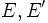$E,E'$ are vector bundles over$M$. Suppose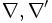$\nabla, \nabla'$ are connections on$E,E'$ respectively. Suppose, further, that the Riemann curvature tensor of$\nabla$ as well as of$\nabla'$ is zero. In other words, both$\nabla$ and$\nabla'$ are flat connections. Then, the tensor product of connections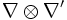$\nabla \otimes \nabla'$ is also a flat connection.

## Facts used

1. Formula for curvature of tensor product of connections: This states that:$R_{\nabla \otimes \nabla'}(X,Y)(s \otimes s') = R_\nabla(X,Y)(s) \otimes s' + s \otimes R_\nabla'(X,Y)(s')$.

## Proof

### Proof using the formula for curvature of tensor product

By the formula for tensor product of connections, we have:$R_{\nabla \otimes \nabla'}(X,Y)(s \otimes s') = R_\nabla(X,Y)(s) \otimes s' + s \otimes R_\nabla'(X,Y)(s')$.

The right side is zero. Thus,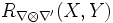$R_{\nabla \otimes \nabla'}(X,Y)$ is identically zero on all pure tensors. Further, since$R$ is a tensor,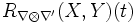$R_{\nabla \otimes \nabla'}(X,Y)(t)$ at a point depends only on the value of$t$ at that point. Thus, at every point, we have shown that$R_{\nabla \otimes \nabla'}(X,Y)$ is identically zero on all pure tensors. Since every tensor is a sum of pure tensors and$R_{\nabla \otimes \nabla'}(X,Y)$ is linear,$R_{\nabla \otimes \nabla'}(X,Y)$ is identically zero at each point, and hence identically zero as a tensor. Thus,$\nabla \otimes \nabla'$ is flat.